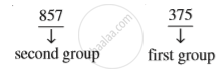Share

# Cube Roots - Cube Root of a Cube Number

Course

#### notes

If you know that the given number is a cube number then following method can be used.
Step 1: Take any cube number say 857375 and start making groups of three digits starting from the right most digit of the number.We can estimate the cube root of a given cube number through a step by step process.
We get 375 and 857 as two groups of three digits each.

Step 2: First group, i.e., 375 will give you the one’s (or unit’s) digit of the required cube root.
The number 375 ends with 5. We know that 5 comes at the unit’s place of a number only when it’s cube root ends in 5.
So, we get 5 at the unit’s place of the cube root.

Step 3: Now take another group, i.e., 857.
We know that 93 = 729 and 103 = 1000. Also, 729 < 857 < 1000. We take the one’s place, of the smaller number 729 as the ten’s place of the required cube root. So, we get $\sqrt{857375}$ = 95.